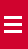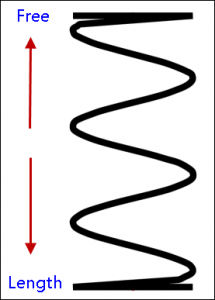# What is meant by free length of a spring?

The free length of a spring is defined as the length of a compression spring without any force or load applied to it.The compression spring undergoes deflection when a force or load is applied to it. Even if the load or force applied is small, the spring may deflect by at least a thousand of an inch. The deflection depends on the amount of force applied, and durability of the spring.If the free length of the compression spring is larger than its diameter, then it is going to knuckle or bend. This is known as a slenderness ratio. For calculating the slenderness ratio, you must first calculate the mean diameter. The mean diameter is the difference between the diameter of a wire and its outer diameter. The value obtained is divided by the free length.  This result is expressed as x:1 (x to 1).